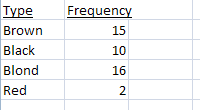# Probability Frequency Distribution: How to Solve Problems in Easy Steps

Share on

Watch the video or read the steps below to learn how to solve a simple problem involving a probability frequency distribution:

Probability frequency distribution questions in statistics always have the term “frequency distribution” in the question. For example, the question might ask you to figure out the probability of a simple event happening, using a frequency distribution table. If you don’t have the phrase “frequency distribution” in the question, see other problem types in the probability index.

## Probability frequency distribution: Overview.

A probability frequency distribution is a way to show how often an event will happen. It also shows what the probability of each event happening is. A frequency distribution table can be created by hand, or you can make a frequency distribution table in Excel.

## Probability frequency distribution: Steps

Sample question: In a sample of 43 students:

Use a frequency distribution table to find the probability a person has neither red nor blond hair.

Step 1: Make a frequency distribution table.
List the items in one column and the number of items in a second column. In this case, your items are hair colors: brown, black, blond, red.Tip: If you have a large number of items, use tally marks to help you find the total.

Step 2: Add up the totals.
In the sample question we’re asked for the odds a person will not have blond or red hair. In other words, we want to know the probability of a person having black or brown hair. Note that you’re told in the question there are 43 students in the class.

Brown = 15/43 (15 out of 43 students have brown hair).
Black = 10/43 (10 out of 43 students have black hair).

Add these together to get the total number of students who have brown or black hair.

15/43 + 10/43 = 25/43 (25 out of 43 students have either brown or black hair).

You’re done with solving this Probability frequency distributi

CITE THIS AS:
Stephanie Glen. "Probability Frequency Distribution: How to Solve Problems in Easy Steps" From StatisticsHowTo.com: Elementary Statistics for the rest of us! https://www.statisticshowto.com/probability-and-statistics/probability-main-index/probability-frequency-distribution/
------------------------------------------------------------------------------

Need help with a homework or test question? With Chegg Study, you can get step-by-step solutions to your questions from an expert in the field. Your first 30 minutes with a Chegg tutor is free!